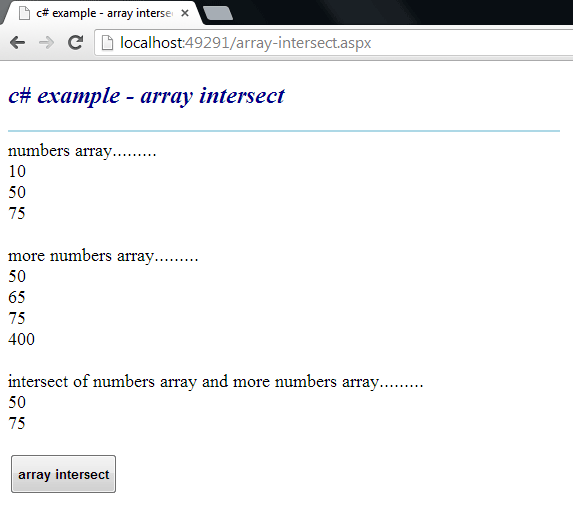### c# - How to intersect two arrays

Intersect two Arrays
The Array class provides methods for creating, manipulating, searching, and sorting arrays. The Array class is not part of the System.Collections namespaces. However, it is still considered a collection because it is based on the IList interface. An element is a value in an Array. The length of an Array is the total number of elements it can contain. The Array has a fixed capacity.

The following .net c# tutorial code demonstrates how we can intersect two array instances. But there is no built-in method in the Array class two intersect two Array objects. So in this .net c# tutorial code, we will use Enumerable Intersect() method to intersect two Array instances. Here we will intersect two integer-type Array instances.

The Enumerable Intersect() method produces the set intersection of two sequences. This method is located under System.Linq namespace. The Enumerable Intersect<TSource>(IEnumerable<TSource>, IEnumerable<TSource>) method overload produces the set intersection of two sequences by using the default equality comparer to compare values.

The Intersect(first, second) method’s first parameter is an IEnumerable<T> whose distinct elements that also appear in the second will be returned. The second parameter is an IEnumerable<T> whose distinct elements that also appear in the first sequence will be returned.

The Enumerable Intersect() method returns a sequence that contains the elements that form the set intersection of two sequences. The Intersect(first, second) method throws ArgumentNullException if the first or second is null.
array-intersect.aspx
``````
<%@ Page Language="C#" AutoEventWireup="true"%>

<!DOCTYPE html>
<script runat="server">
protected void Button1_Click(object sender, System.EventArgs e)
{
int[] numbers = { 10,50,75};
int[] morenumbers = { 50,65,75,400};

Label1.Text = "numbers array.........<br />";
foreach (int i in numbers)
{
Label1.Text += i.ToString() + "<br />";
}

Label1.Text += "<br />more numbers array.........<br />";
foreach (int i in morenumbers)
{
Label1.Text += i.ToString() + "<br />";
}

var intersect = numbers.Intersect(morenumbers);

Label1.Text += "<br />intersect of numbers array and more numbers array.........<br />";
foreach (int i in intersect)
{
Label1.Text += i.ToString() + "<br />";
}
}
</script>

<html xmlns="http://www.w3.org/1999/xhtml">
<title>c# example - array intersect</title>
<body>
<form id="form1" runat="server">
<div>
<h2 style="color:DarkBlue; font-style:italic;">
c# example - array intersect
</h2>
<hr width="550" align="left" color="LightBlue" />

<asp:Label
ID="Label1"
runat="server"
Font-Size="Large"
>
</asp:Label>
<br />
<asp:Button
ID="Button1"
runat="server"
Text="array intersect"
OnClick="Button1_Click"
Height="40"
Font-Bold="true"
/>
</div>
</form>
</body>
</html>
``````More c# examples